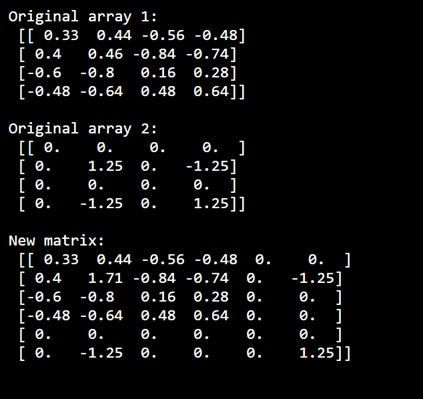# How to add a 4x4 matrix values into a 6x6 matrix using numpy?

Learn, How to add a 4x4 matrix values into a 6x6 matrix using numpy?
Submitted by Pranit Sharma, on January 01, 2023

NumPy is an abbreviated form of Numerical Python. It is used for different types of scientific operations in python. Numpy is a vast library in python which is used for almost every kind of scientific or mathematical operation. It is itself an array which is a collection of various methods and functions for processing the arrays.

## Adding a 4x4 matrix values into a 6x6 matrix

However, adding two arrays of different dimensions is a little bit tricky with NumPy but, with array comprehension, we can do it in such a way that if we have two matrices m1 and m2, we will create two temporary arrays m3 and m4 from m1 and m2 respectively. Then finally we will create a final array m5 which would be the resulting array.

Let us understand with the help of an example,

## Python code to add a 4x4 matrix values into a 6x6 matrix using numpy

```# Import numpy
import numpy as np

# Creating two numpy array of 4x4
a1 = np.array([[ 0.33,  0.44,  -0.56,  -0.48],
[ 0.4,  0.46,  -0.84,  -0.74],
[ -0.6, -0.8, 0.16, 0.28],
[-0.48, -0.64, 0.48, 0.64]])

a2 = np.array([[ 0,  0,  0,  0],
[ 0,  1.25,  0,  -1.25],
[ 0,  0,  0,  0],
[ 0,  -1.25,  0,  1.25]])

# Display original arrays
print("Original array 1:\n",a1,"\n")
print("Original array 2:\n",a2,"\n")

# Creating temporary matrices of 6X6
a3, a4 = np.zeros((6, 6)), np.zeros((6, 6))

# Rearranging values for a3 with a1 and a3 with a2
a3[0:4, 0:4] = a1[0:4, 0:4] + a3[0:4, 0:4]
a4[0:2, 0:2] = a2[0:2, 0:2]
a4[0:2, 4:6] = a2[0:2, 2:4]
a4[4:6, 0:2] = a2[2:4, 0:2]
a4[4:6, 4:6] = a2[2:4, 2:4]

# Creating final array
res = a3+a4

# Display result
print("New matrix:\n",res,"\n")
```

Output: